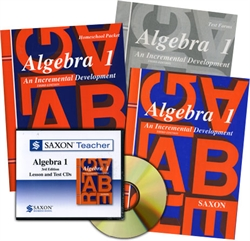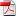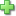# Saxon Algebra 1 - Home School Bundle with Teacher CD

Publisher: Saxon Publishers
3rd Edition, Item: 33110
Curriculum Bundle
List Price: \$218.70 Our Price: \$196.84

See series description for full review.

Algebra 1 covers topics typically treated in a first-year algebra course. Specific topics include exponents and roots, properties of real numbers, absolute value, scientific notation, polynomials and rational expressions, Pythagorean theorem, algebraic proofs, functions, quadratic equations, exponential growth, computation of the perimeter and area of two dimensional regions, computation of the surface area and volume of a wide variety of geometric solids, statistics and probability, and more. (120 lessons) The Home Study Kit, which contains the student textbook, answer key and test packet, and the Saxon Teacher CD (which contains lecture CDs and the complete solutions) are both included in this bundle, with a 15% discount.

## Saxon Samples:Text Table of ContentsLesson 1Lesson 16Lesson 27Lesson 31Lesson 32Lesson 73Lesson 83 page 1Lesson 83 page 2Test 20Click here to write a review